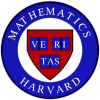Summer Tutorials 2003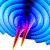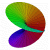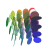NOTE: This is an archived page from a previous tutorial. Information about the current tutorials can be found here.

 Welcome Message
Greetings,

I am writing to officially announce the 2003 version of our Summer Tutorial program. The idea here is to offer you some interesting mathematics if you have an ulterior motive for being in the Boston area during July and the beginning of August. In particular, the tutorials will run for six weeks starting roughly at the beginning of July, meeting twice per week in the evenings (so as not to interfere with day time jobs). The precise starting dates and meeting times will be arranged for the convenience of the participants once the tutorial rosters are set.

The format will be much like that of the term time tutorials, with the tutorial leader lecturing in the first few meetings and students lecturing later on. Here, I should say that these tutorials have no official Harvard status, so you won't receive either Harvard or concentration credit for them. Moreover, enrollment in the tutorial does not qualify you for any Harvard related perks (such as a place to live). However, the Math Department will pay each student participant a stipend of \$700 and you can hand in your final paper from the tutorial for you junior 5-page paper requirement.

If there is sufficient interest, we will be offering 5 tutorials this summer; the topics and leaders are as follows:

 'Symplectic and Contact Geometry' lead by Ciprian Manolescu. 'Real and Complex Manifolds' lead by Deepee Khosla. 'Topics in Group Theory' lead by Abhinav Kumar. 'Geometry and General Relativity' lead by Vivek Mohta. 'Topics in Topological Graph Theory' lead by Trevor Bass.

Below, you will find a brief description of each tutorial topic. Moreover, the tutorial leaders will describe their tutorials at the April 8 Math Table.
You can sign up for a tutorial by emailing your desire to me at chtaubes@math.harvard.edu. When you do sign up, please list at least one other choice in case your desired tutorial is either over subscribed or under subscribed.
What follows are some brief descriptions of the tutorial topics. If you have further questions about any given topic, contact the tutorial leader via the email address given below. If you have questions about the administration of the tutorials, ask me.

-- Cliff Taubes

 Tutorial: 'Symplectic and Contact Geometry'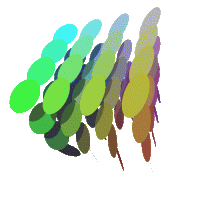A space can be endowed with various kinds of geometric structures: a real differentiable structure describes a manifold, a complex structure describes a complex manifold, and a Riemannian structure describes a manifold together with a metric. An important notion coming from physics is that of a symplectic structure, which describes the phase space of a mechanical system. Symplectic manifolds have also been at the center of recent developments in quantum physics, such as string theory. However, due to the less intuitive character of a symplectic structure, it took mathematicians a long time to answer some basic questions about symplectic manifolds: a famous example is the nonsqueezing theorem (one cannot squeeze a ball into a cylinder of smaller base radius by a symplectic transformation), which was proved by Gromov in 1985. This tutorial will serve as an introduction to the world of symplectic manifolds and their odd-dimensional counterpart, contact manifolds. Prerequisites: A solid knowledge of manifolds, differential forms, and vector fields, at the level of Math 25/55 or 135. For further information, see the tutorial webpage or contact Ciprian Manolescu at manolesc@fas.

 Tutorial: 'Real and Complex Manifolds'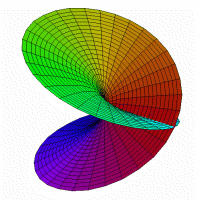Real and complex manifolds are the basic objects of modern geometry. In this tutorial, we will explore the interrelations between topology, differential geometry, and algebraic geometry. In the first part of the course, we will explore smooth (or real) manifolds. One of the central themes will be how notions from topology can be locally encoded on a manifold using calculus. For example, the Euler characteristic of a manifold can be computed by suitably integrating the curvature form over the whole manifold. A complex manifold is just a real manifold with the additional specifiction of a local complex structure around each point. This little extra data really gives a much richer structure. For example, the set of all complex structures on a given smooth manifold can itself be made into a complex manifold; this is an example of a moduli space. In many important cases, a complex structure is "the same" as an algebraic structure, and this allows to apply differential methods to the study of algebraic varieties. By the end of the course, topology, geometry, and algebra will converge. For example, we will derive relations between elliptic functions using knowledge of the topology of a plane cubic in the complex projective plane. We will see how diffential forms are a tool that can simultaneously probe cohomology and moduli---this is the beginning of Hodge theory. Throughout the tutorial, we'll focus on specific examples to illustrate the theory we develop. We'll pay special attention to Lie groups as examples of real manifolds and Riemann surfaces (a.k.a. algebraic curves) as examples of complex manifolds. The student should have a good knowledge of mulitvariable calculus and linear algebra as well as real and complex analysis. Acquaintance with some algebraic topology would be helpful but is not required. For additional information, contact Deepee Khosla deepee@math

 Tutorial: 'Topics in Group Theory'This tutorial will discuss selected topics in group theory and some of their exciting applications. We will first develop some basic structure theory of groups, introducing concepts such as the Sylow subgroups, solvability and simplicity. Then we will start looking at examples of groups that arise in different situations and use these techniques to discover their properties. The results we will prove include the simplicity of the alternating group An for n >4, the simplicity of the Mathieu groups (which are sporadic simple groups) and the Nielsen Schreier theorem (every subgroup of a free group is free). Among the groups we will run into are Coxeter groups, classical groups over finite fields, the group of Moebius transformations and even the Rubik's cube group. Near the end of the course, we will try to get an understanding of the monster simple group and outline some of the remarkable mathematics it has generated. Prerequisites: Some basic knolwdge of group theory and linear algebra. We will give an outline of the properties of the fundamental group and of representations, if needed. Note: The dates for the tutorial will be June 30 to August 8. For additional information, contact Abhinav Kumar abhinav@math

 Tutorial: 'Geometry and General Relativity'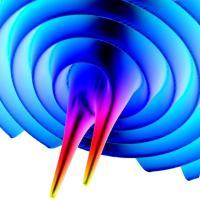You've heard about black holes, maybe in physics classes or maybe on "Star Trek". In this tutorial, we'll develop the geometric tools to understand them at a deeper level. We'll start with (Riemannian) geometry in two spatial dimensions to develop some basic intuition about concepts like curvature and geodesics. We'll continue to (Riemannian) geometry in three spatial dimensions and then to (semi-Riemannian) geometry in two spatial and one temporal dimensions. An important aspect of the course will be understanding the difference between these two settings. Finally, we'll move to the real world with three spatial and one temporal dimension. This will be the language in which we motivate and write down Einstein's equations of general relativity. The rest of the tutorial will be the solution of these in various contexts and at various levels of approximation. For example, we will calculate the deflection of star-light by the sun and the precession of the semi-major axis of Mercury's orbit, two of the early experimental tests of general relativity. The culmination of the tutorial will be the black hole solutions of Einstein's equations. We'll understand what horizons are and what happens to the unfortunate astronauts that venture inside them. Prerequisites: Knowledge of linear algebra and differential equations at the level of Math 21b, two 100-level math courses, and knowledge of physics at the level of Physics 15. For further information, please contact Vivek Mohta (vmohta@math).

 Tutorial: 'Topics in Topological Graph Theory'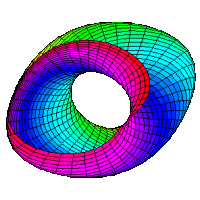Topological graph theory has been around for over a century, but in the last couple of decades there has been a huge surge of interest as a few of the major outstanding problems in the field were solved in a groundbreaking series of 22 papers by Robertson and Seymour, which introduced what is called "excluded minor theory." Since then, most people in the field have switched over to studying the theory put forth by these papers, and the applications of topological graph theory to topology, algorithms, complexity theory, and other areas grew enormously. In the tutorial we will first present basic results in surface topology (at a very basic level), graph theory, and topological graph theory. We will offer proofs of the graph-theoretic classification of 2-manifolds, Kuratowski's theorem, and other elementary embedding results. We will then move on to coloring problems, such as the four-color theorem and its generalization to higher surfaces. We will then discuss major theorems of excluded minor theory, including a generalization of Kuratowski's theorem that is the gem of Robertson-Seymour theory: given any infinite set of graphs, there exist two such that one is a minor of the other (the graph minor theorem). We will prove the excluded minor theorem, and present the theory of well-quasi-ordering to make papers in the field better understandable. Finally we will discuss applications in complexity theory (and provide a brief introduction to theoretical computer science, assuming no background). The problem of determining the minimum genus of a surface on which a graph can be embedded is NP-complete, but there are many partial results, including a recent linear-time algorithm that given a surface, decides whether an arbitrary graph is embeddable. Approximate schedule: Lectures 1-3: Basic results in topology, graph theory, topological graph theory. Proofs of the graph-theoretic classification of 2-manifolds, Kuratowski's theorem, and other elementary embedding results. Lecture 4-5: Coloring problems, such as the four-color theorem and its generalization to higher surfaces (the generalization is much easier and was proved long ago). Lectures 6-8: The major theorems of excluded minor theory, including a generalization of Kuratowski's theorem that is the gem of Robertson-Seymour theory: given any infinite set of graphs, there exist two such that one is a minor of the other (the graph minor theorem). We will also prove the excluded minor theorem, which I prove in my thesis, and present the theory of well-quasi-ordering. Lectures 9-10: Applications to computer science. The problem of determining the minimum genus of a surface on which a graph can be embedded is NP-complete, but there are many partial results, including a recent linear-time algorithm that given a surface, decides whether an arbitrary graph is embeddable. Presentations: There is an amazing wealth of material students could present, especially as this tutorial will be by its very nature surveyish in nature. We will be discussing two monstrous results, the four color theorem and the graph minor theorem, the former of which has several books written about it and the latter of which will once people are able to better condense the proof. Thus there are a lot of partial and auxiliary results students could present on. For those more interested in topology or computer science, there are many papers in these areas that will be made readibly accessible by the tutorial. Prerequisites: A knowledge of how to write proofs. (If the students in the class know basic topology and/or basic graph theory, we will skip over some introductory stuff.) To get more information, contact Trevor Bass (tbass@fas).

 Archive: Old Summer Tutorials since 2001

Last update, 3/21/2003